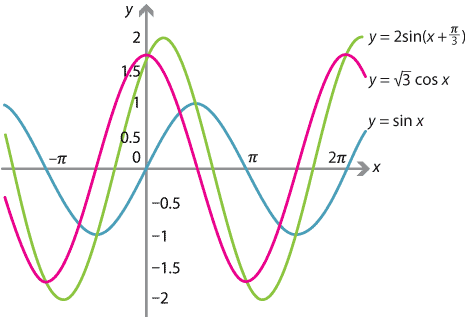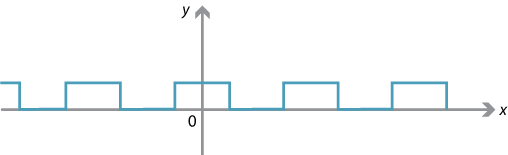We can add together a sine and a cosine curve. Their sum can be obtained graphically by adding the $$y$$-values of the two curves. It turns out that, if the waves have the same period, this will produce another trigonometric graph with a change in amplitude and a phase shift. Physically, this is called superimposing one wave with another.

This can also be done algebraically. For example, to add $$\sin x$$ and $$\sqrt{3}\cos x$$, we proceed as follows. Divide their sum by $$\sqrt{1^2 + (\sqrt{3})^2} = 2$$, so

$\sin x + \sqrt{3}\cos x = 2\Biggl(\dfrac{1}{2}\sin x + \dfrac{\sqrt{3}}{2}\cos x\Biggr).$

This looks a little like the expansion $$\sin(x+ \alpha) = \sin x\,\cos \alpha + \cos x\,\sin \alpha$$. We thus equate $$\cos \alpha = \dfrac{1}{2}$$ and $$\sin \alpha = \dfrac{\sqrt{3}}{2}$$, which implies that $$\alpha = \dfrac{\pi}{3}$$. Hence

$\sin x + \sqrt{3}\cos x = 2\sin\Bigl(x+ \dfrac{\pi}{3}\Bigr).$

The new wave has amplitude 2 and a phase shift of $$\dfrac{\pi}{3}$$ to the left.In general, the same method will work to add $$a\sin x + b\cos x$$, in which case we divide out by $$\sqrt{a^2+b^2}$$.

Exercise 19

Express $$3\cos x - 4\sin x$$ in the form $$A\sin(x+\alpha)$$.

Waves such as the square wave and the saw-tooth wave, which arise in physics and engineering, can be approximated using the sum of a large number of waves — this is the study of Fourier series, which is central to much of modern electrical engineering and technology.

The following square wave is the graph of the function with rule

$\dfrac{1}{2} + \dfrac{2}{\pi}\cos x - \dfrac{2}{3\pi}\cos 3x + \dfrac{2} {5\pi}\cos 5x - \dotsb = \dfrac{1}{2} + \dfrac{2}{\pi}\sum_{n=1}^\infty \dfrac{\sin \dfrac{n\pi}{2}}{n}\cos nx.$Next page - Links forward - The t formulas